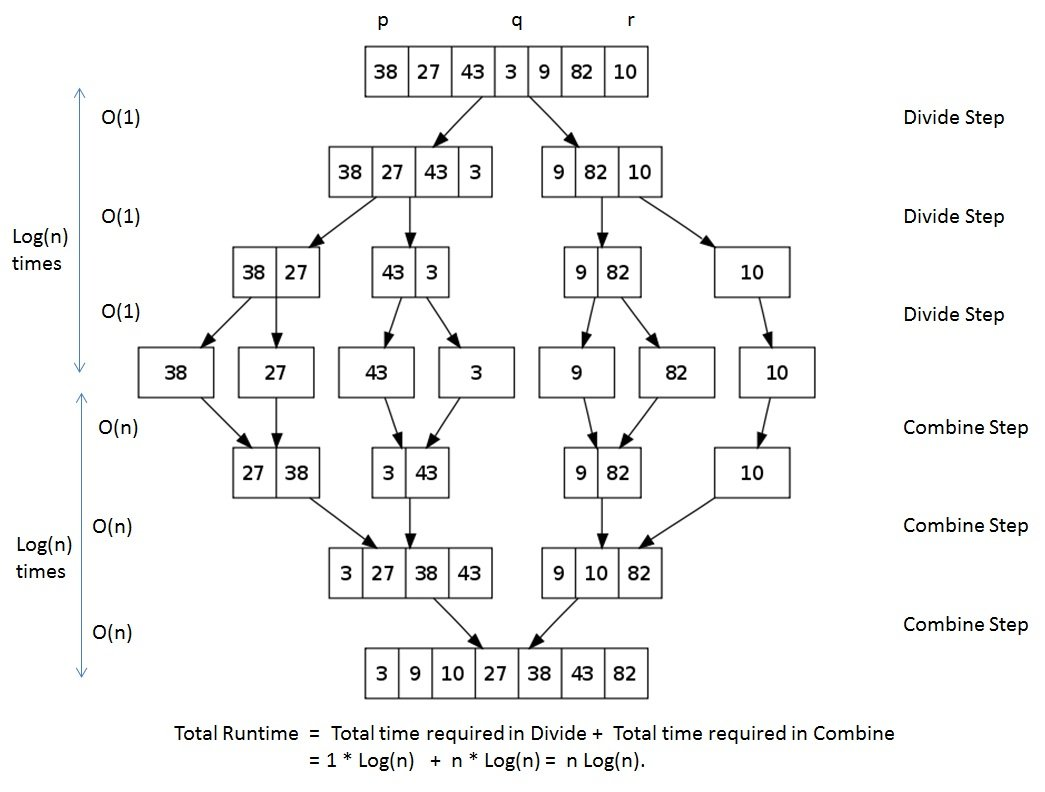## Merge Sort

Similar to Quicksort, Merge sort is a divide and conquer algorithm that generally performs better than quadratic sorting algorithms such as bubble sort, insertion sort and selection sort. But unlike these other sorts, which take list and modify it in place, merge sort requires additional space to merge 2 lists together.

## How Merge Sort Works

• 1) Divide the unsorted array into n partitions, each partition contains 1 element. Here the one element is considered as sorted.
• 2) Repeatedly merge partitioned units to produce new sublists until there is only 1 sublist remaining. This will be the sorted list at the end.## Merge Sort Complexity

Merge sort is a fast, stable sorting routine that is O(n log n). When sorting arrays, merge sort requires additional temporary space proportional to the size of the input array. Merge sort is relatively simple to code and offers performance that is almost as good as quicksort.

## Merge Sort Algorithm

Below is an example of Merge Sort written in Java..

```package miftyisbored;

/**
* @author m_yusuf
*	This is a small class that implements merge Sort
*/
public class MiftyMergeSorter {
/**
* This main method is used to illustrate how to use the Merge Sort Algorithm
**/
public static void main(String[ ] args)
{
final String BLANK_SPACE = "  "; // Just because I am lazy...
int[ ] data = { 10000, 1002, 83, 12, 58, 72, 66, 65, 75648, 90, 23, 37, 41, 66, 0, 3100, 55555, 1, 67 };

// Print the array before sorting:
System.out.println("Welcome to Mifty's Merge Sorter");
System.out.println("Here is the original unsorted list:");
for (int i = 0; i < data.length; i++)
System.out.print(data[i] + BLANK_SPACE);
System.out.println( );

// Sort the numbers, and print the results
mergeSort(data);
System.out.println("All numbers are sorted now!");
System.out.println("Here is the ordered list:");
for (int i = 0; i < data.length; i++)
System.out.print(data[i] + BLANK_SPACE);
System.out.println( );
}

/**
* This function sorts an array of integers from smallest to largest using merge sort
* @param: data - the array to be sorted
*/
private static void mergeSort(int[ ] data){
int length = data.length;
if(length < 2) return;

int middle = length / 2;

// create and populate the left array
int[] leftData = new int[middle];
for(int i=0;i<middle;i++){
leftData[i] = data[i];
}

// create and populate the left array
int[] rightData = new int[length - middle];
for(int i=middle;i<length;i++){
rightData[i-middle] = data[i];
}

// recursively call ourself and merge
mergeSort(leftData);
mergeSort(rightData);
merge(leftData,rightData,data);
}

/**
* This function merges two arrays into a master array
* @param: first - the first array
* @param: second - the second array
* @param: data - the merged array
*/
private static void merge(int[ ] first, int[ ] second, int[ ] merge){
int firstLength = first.length;
int secondLength = second.length;
int firstIndex =0;
int secondIndex=0;
int mergeIndex=0;

while( firstIndex < firstLength && secondIndex < secondLength){
if(first[firstIndex] <= second[secondIndex]){
merge[mergeIndex] = first[firstIndex];
firstIndex++;
}else{
merge[mergeIndex] = second[secondIndex];
secondIndex++;
}
mergeIndex++;
}

// check for leftovers in first array
while(firstIndex < firstLength){
merge[mergeIndex] = first[firstIndex];
firstIndex++;
mergeIndex++;
}

// check for leftovers in second array
while(secondIndex < secondLength){
merge[mergeIndex] = second[secondIndex];
secondIndex++;
mergeIndex++;
}
}
}
```

The following is the output of the application

```Welcome to Mifty's Merge Sorter
Here is the original unsorted list:
10000  1002  83  12  58  72  66  65  75648  90  23  37  41  66  0  3100  55555  1  67
All numbers are sorted now!
Here is the ordered list:
0  1  12  23  37  41  58  65  66  66  67  72  83  90  1002  3100  10000  55555  75648

```

Mifty Yusuf is a Montreal-based software developer who enjoys playing with new web technologies as well as comic books and illustrations. He beleives that, no matter what the question is, the answer is always Batman!

Go to Top